# Lectures on Power System Analysis 01 Dr Kassim

• Slides: 25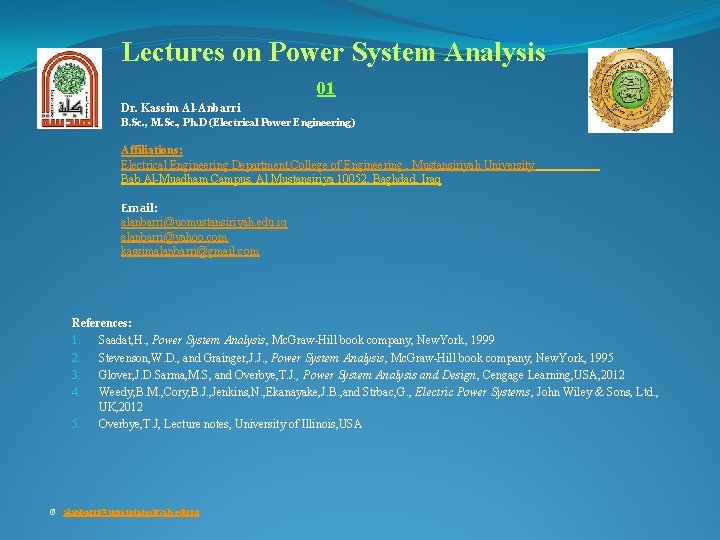Lectures on Power System Analysis 01 Dr. Kassim Al-Anbarri B. Sc. , M. Sc. , Ph. D (Electrical Power Engineering) Affiliations: Electrical Engineering Department, College of Engineering , Mustansiriyah University Bab Al-Muadham Campus, Al Mustansiriya 10052, Baghdad, Iraq Email: [email protected] edu. iq [email protected] com [email protected] com References: 1. Saadat, H. , Power System Analysis, Mc. Graw-Hill book company, New. York, 1999 2. Stevenson, W. D. , and Grainger, J. J. , Power System Analysis, Mc. Graw-Hill book company, New. York, 1995 3. Glover, J. D. Sarma, M. S, and Overbye, T. J. , Power System Analysis and Design, Cengage Learning, USA, 2012 4. Weedy, B. M. , Cory, B. J. , Jenkins, N. , Ekanayake, J. B. , and Strbac, G. , Electric Power Systems, John Wiley & Sons, Ltd. , UK, 2012 5. Overbye, T. J, Lecture notes, University of Illinois, USA © [email protected] edu. iq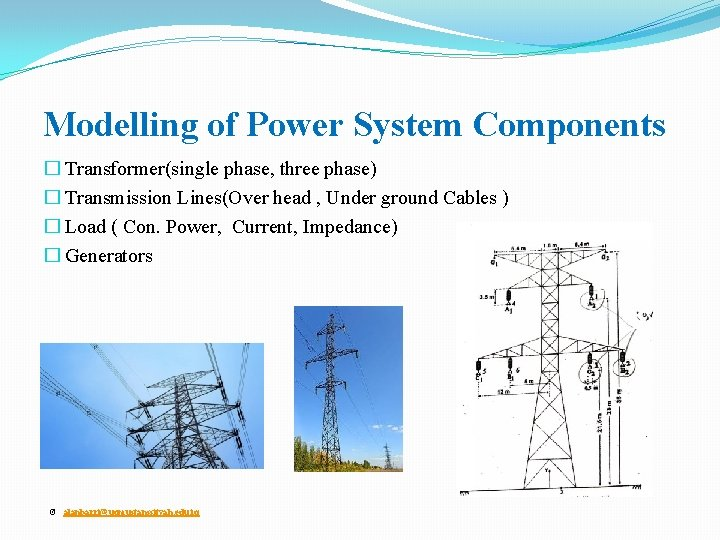Modelling of Power System Components � Transformer(single phase, three phase) � Transmission Lines(Over head , Under ground Cables ) � Load ( Con. Power, Current, Impedance) � Generators © [email protected] edu. iq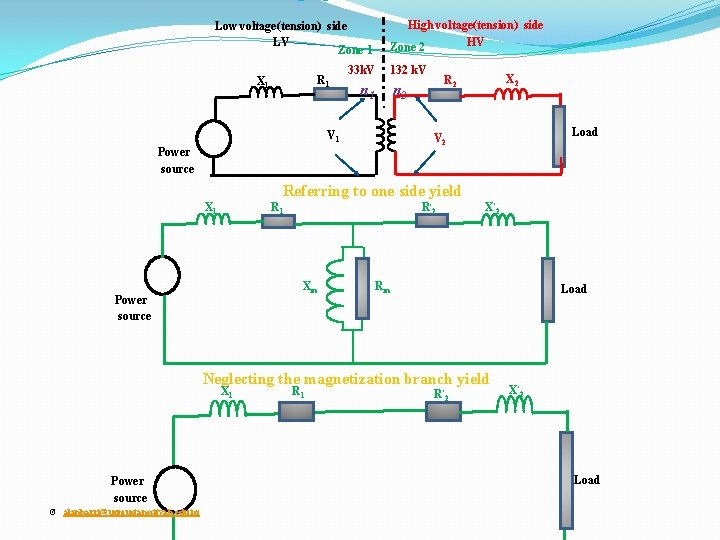Low voltage(tension) side LV Zone 1 R 1 X 1 High voltage(tension) side HV Zone 2 33 k. V 132 k. V V 1 X 2 R 2 n 1 n 2 Load V 2 Power source Referring to one side yield X 1 R 1 R ׳ 2 Xm Power source X ׳ 2 Rm Load Neglecting the magnetization branch yield Power source © [email protected] edu. iq X 1 R ׳ 2 X ׳ 2 Load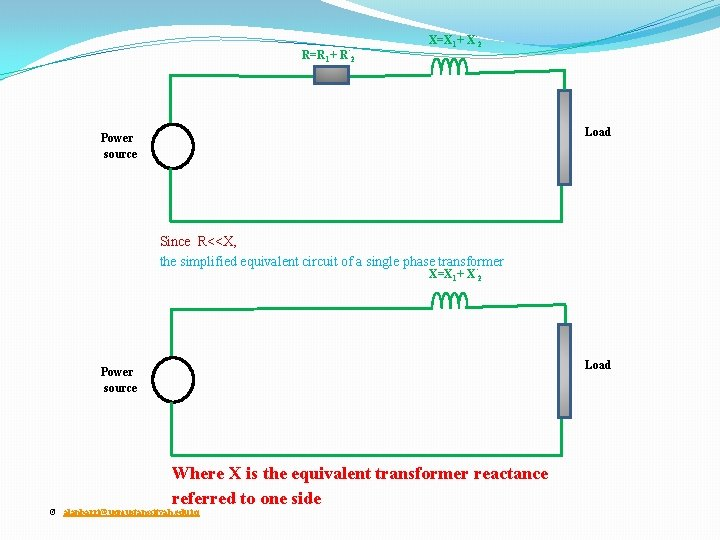R=R 1+ R’ 2 X=X 1+ X’ 2 Load Power source Since R<<X, the simplified equivalent circuit of a single phase transformer X=X 1+ X’ 2 Load Power source Where X is the equivalent transformer reactance referred to one side © [email protected] edu. iqTransmission Line π model L VS Sending end voltage C/2 R C/2 VR Receiving end voltage R= Resistance of the line = Length of line * resistance per unit length L= Inductance of the line = Length of line * inductance per unit length C= Capacitance of the line = Length of line * capacitance per unit length For short lines <50 km , Capacitance and resistance are neglected © [email protected] edu. iq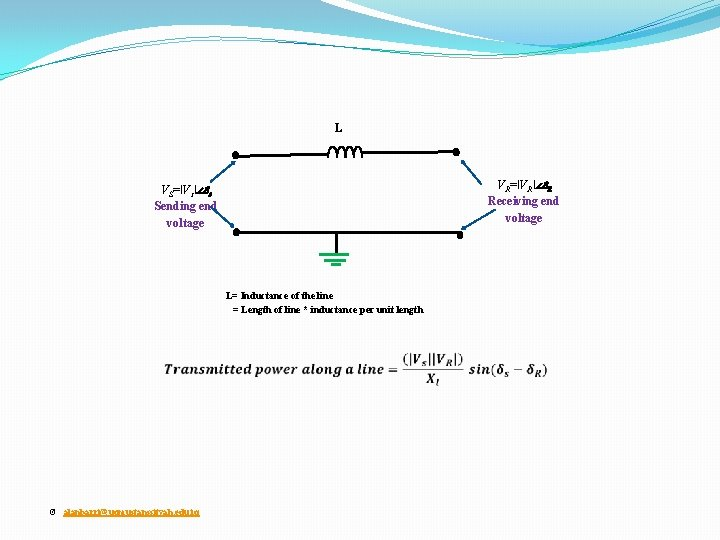L VR=ǀVRǀ∠δR Receiving end voltage VS=ǀVsǀ∠δs Sending end voltage L= Inductance of the line = Length of line * inductance per unit length © [email protected] edu. iqI + E 60 V © [email protected] edu. iq R 12 Ω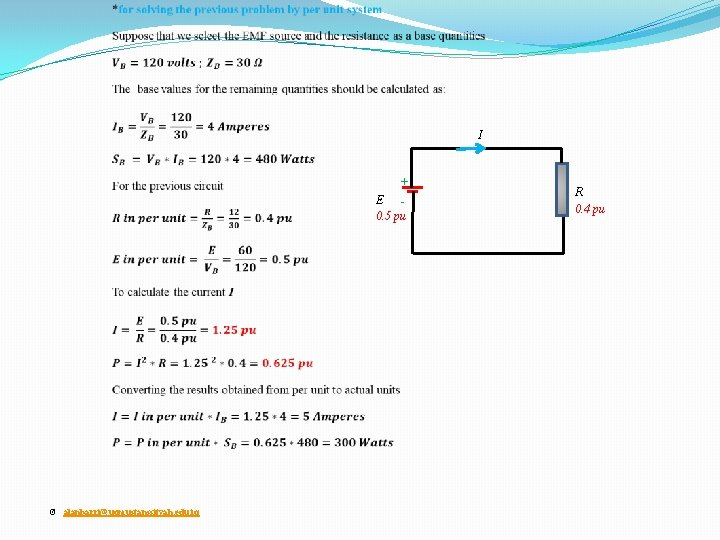I + E 0. 5 pu © [email protected] edu. iq R 0. 4 pu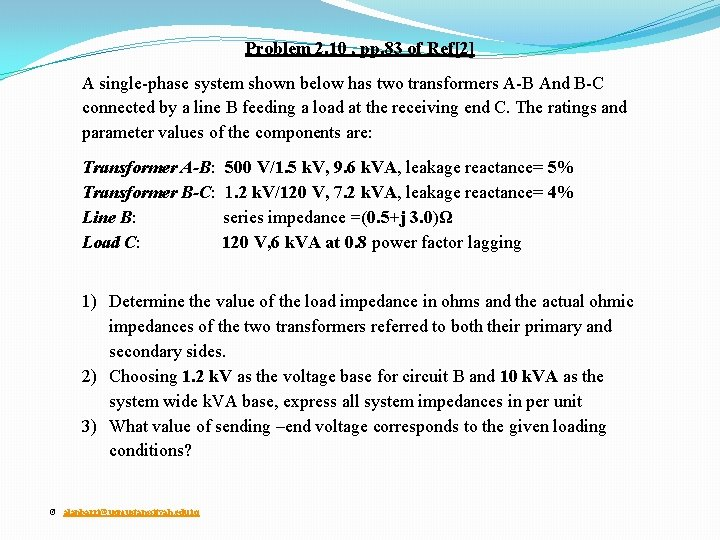Problem 2. 10 , pp. 83 of Ref A single-phase system shown below has two transformers A-B And B-C connected by a line B feeding a load at the receiving end C. The ratings and parameter values of the components are: Transformer A-B: 500 V/1. 5 k. V, 9. 6 k. VA, leakage reactance= 5% Transformer B-C: 1. 2 k. V/120 V, 7. 2 k. VA, leakage reactance= 4% Line B: series impedance =(0. 5+j 3. 0)Ω Load C: 120 V, 6 k. VA at 0. 8 power factor lagging 1) Determine the value of the load impedance in ohms and the actual ohmic impedances of the two transformers referred to both their primary and secondary sides. 2) Choosing 1. 2 k. V as the voltage base for circuit B and 10 k. VA as the system wide k. VA base, express all system impedances in per unit 3) What value of sending –end voltage corresponds to the given loading conditions? © [email protected] edu. iq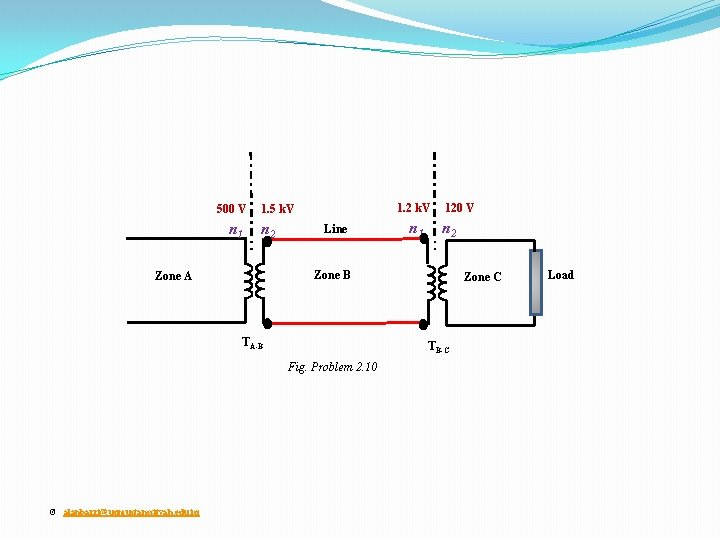1. 2 k. V 120 V 500 V 1. 5 k. V n 1 n 2 Line Zone B Zone A TA-B Zone C TB-C Fig. Problem 2. 10 © [email protected] edu. iq n 1 n 2 Load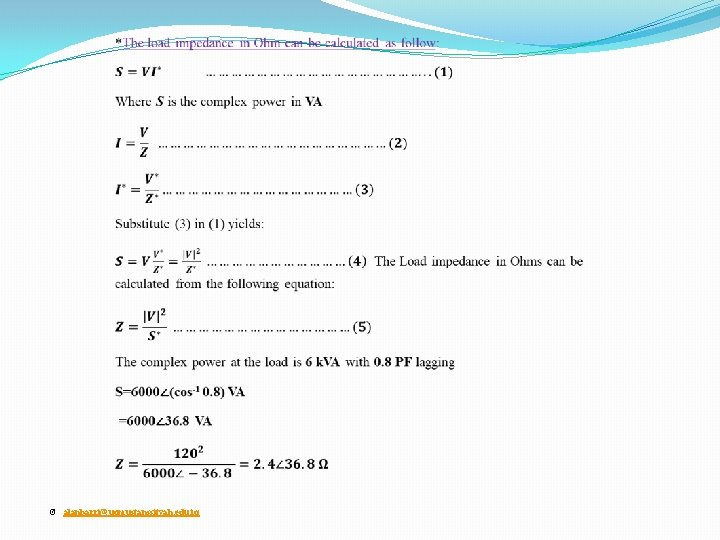500 V 1. 5 k. V n 1 n 2 1 3 Zone A Zone B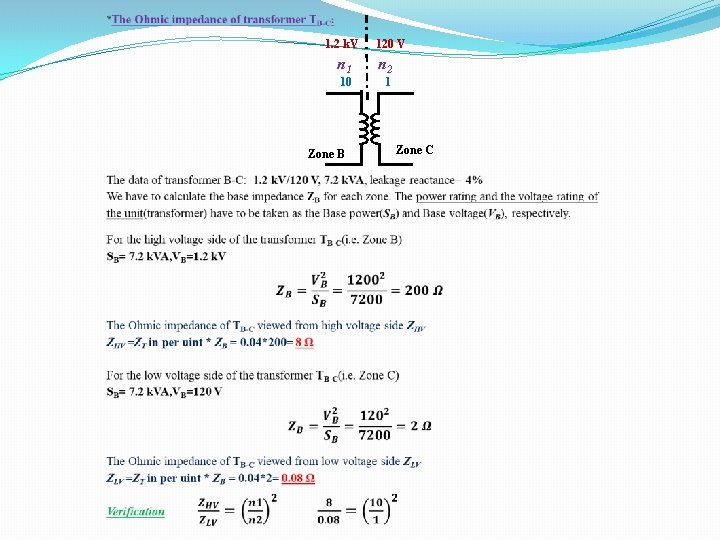1. 2 k. V 120 V n 1 n 2 10 1 Zone B Zone C500 V 1. 5 k. V ZLine n 1 n 2 0. 5+j 3. 0 Ω Zone A © [email protected] edu. iq Zone B TA-B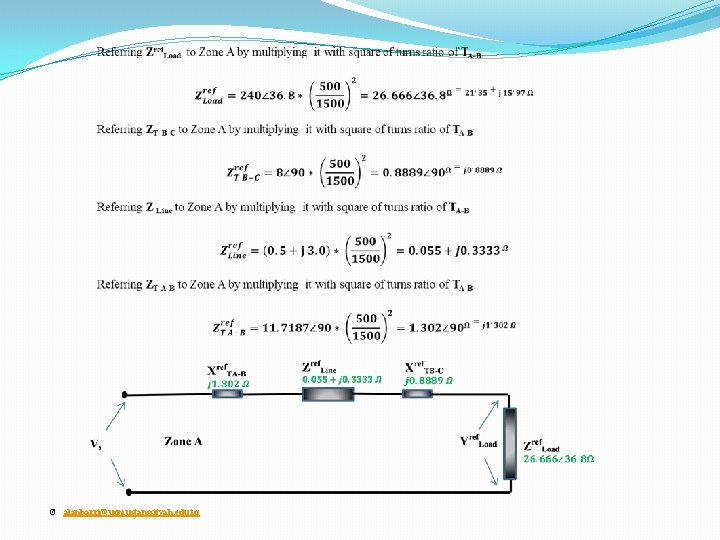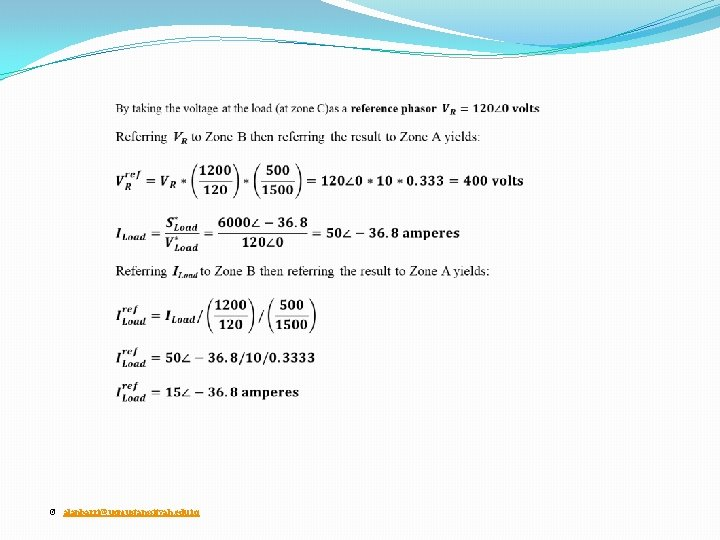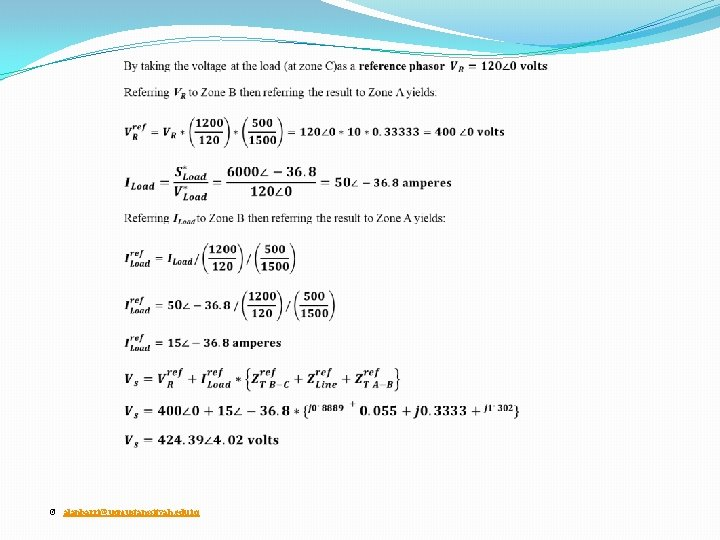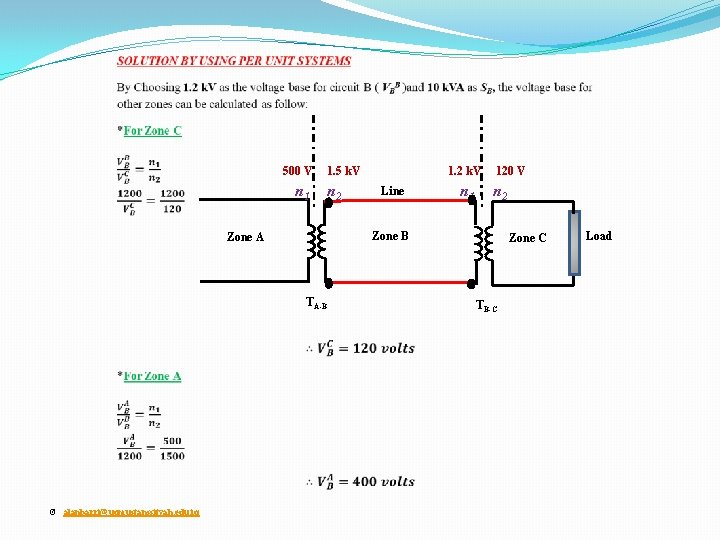1. 2 k. V 120 V 500 V 1. 5 k. V n 1 n 2 Zone B Zone A TA-B © [email protected] edu. iq Line Zone C TB-C Load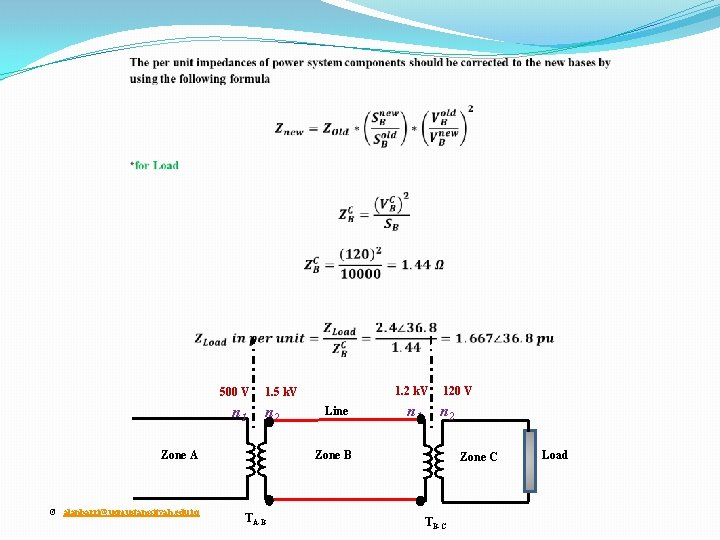1. 2 k. V 120 V 500 V 1. 5 k. V n 1 n 2 Zone B Zone A © [email protected] edu. iq Line TA-B Zone C TB-C Load1. 2 k. V 120 V 500 V 1. 5 k. V n 1 n 2 Zone B Zone A TA-B © [email protected] edu. iq Line Zone C TB-C Load1. 2 k. V 120 V 500 V 1. 5 k. V n 1 n 2 Zone B Zone A TA-B © [email protected] edu. iq Line Zone C TB-C Load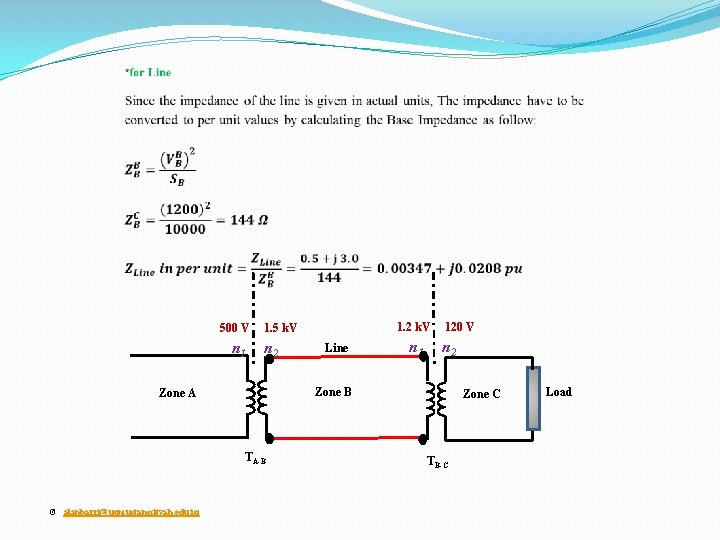1. 2 k. V 120 V 500 V 1. 5 k. V n 1 n 2 Zone B Zone A TA-B © [email protected] edu. iq Line Zone C TB-C Load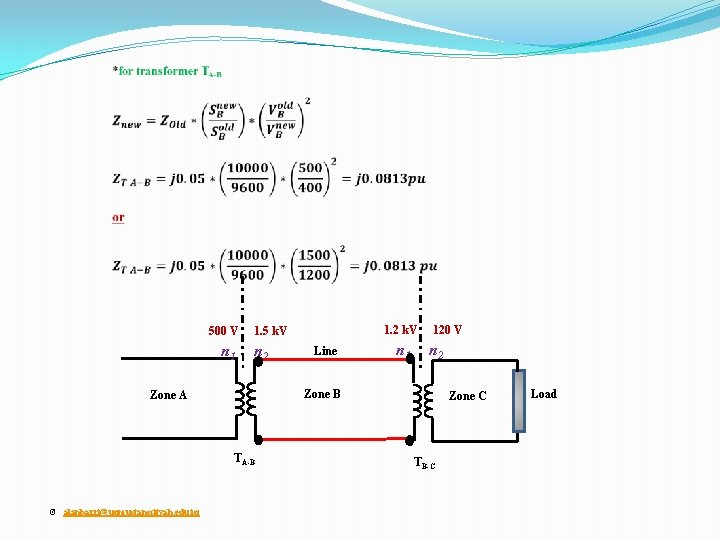1. 2 k. V 120 V 500 V 1. 5 k. V n 1 n 2 Zone B Zone A TA-B © [email protected] edu. iq Line Zone C TB-C Load